true

Take Class 12 Tuition from the Best Tutors

•Affordable fees
•1-1 or Group class
•Flexible Timings
•Verified Tutors

Search inSubrat Majhi
06/09/2018 00

Entropy, the measure of a system’s thermal energy per unit temperaturethat is unavailable for doing useful work. Because work is obtained from ordered molecular motion, the amount of entropy is also a measure of the molecular disorder, or randomness, of a system. The concept of entropy provides deep insight into the direction of spontaneous change for many everyday phenomena. Its introduction by the German physicist Rudolf Clausius in 1850 is a highlight of 19th-century physics.

The idea of entropy provides a mathematical way to encode the intuitive notion of which processes are impossible, even though they would not violate the fundamental law of conservation of energy. For example, a block of ice placed on a hot stove surely melts, while the stove grows cooler. Such a process is called irreversible because no slight change will cause the melted water to turn back into ice while the stove grows hotter. In contrast, a block of ice placed in an ice-water bath will either thaw a little more or freeze a little more, depending on whether a small amount of heat is added to or subtracted from the system. Such a process is reversible because only an infinitesimal amount of heat is needed to change its direction from progressive freezing to progressive thawing. Similarly, compressed gasconfined in a cylinder could either expand freely into the atmosphere if a valve were opened (an irreversible process), or it could do useful work by pushing a moveable piston against the force needed to confine the gas. The latter process is reversible because only a slight increase in the restraining force could reverse the direction of the process from expansion to compression. For reversible processes the system is in equilibrium with its environment, while for irreversible processes it is not.

To provide a quantitative measure for the direction of spontaneous change, Clausius introduced the concept of entropy as a precise way of expressing the second law of thermodynamics. The Clausius form of the second law states that spontaneous change for an irreversible process in an isolated system (that is, one that does not exchange heat or work with its surroundings) always proceeds in the direction of increasing entropy. For example, the block of ice and the stove constitute two parts of an isolated system for which total entropy increases as the ice melts.

By the Clausius definition, if an amount of heat Q flows into a large heat reservoir at temperature Tabove absolute zero, then the entropy increase is ?S = Q/T. This equation effectively gives an alternate definition of temperature that agrees with the usual definition. Assume that there are two heat reservoirs R1 and R2 at temperatures T1 and T2 (such as the stove and the block of ice). If an amount of heat Qflows from R1 to R2, then the net entropy change for the two reservoirs is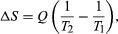which is positive provided that T1 > T2. Thus, the observation that heat never flows spontaneously from cold to hot is equivalent to requiring the net entropy change to be positive for a spontaneous flow of heat. If T1 = T2, then the reservoirs are in equilibrium, no heat flows, and ?S = 0.

The condition ?S ? 0 determines the maximum possible efficiency of heat engines—that is, systems such as gasoline or steam engines that can do work in a cyclic fashion. Suppose a heat engine absorbs heat Q1 from R1and exhausts heat Q2 to R2 for each complete cycle. By conservation of energy, the work done per cycle is W= Q1 – Q2, and the net entropy change is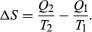To make W as large as possible, Q2should be as small as possible relative to Q1. However, Q2 cannot be zero, because this would make ?Snegative and so violate the second law. The smallest possible value of Q2corresponds to the condition ?S = 0, yielding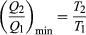as the fundamental equation limiting the efficiency of all heat engines. A process for which ?S = 0 is reversible because an infinitesimal change would be sufficient to make the heat engine run backward as a refrigerator.

The same reasoning can also determine the entropy change for the working substance in the heat engine, such as a gas in a cylinder with a movable piston. If the gas absorbs an incremental amount of heat dQ from a heat reservoir at temperature T and expands reversibly against the maximum possible restraining pressure P, then it does the maximum work dW = PdV, where dV is the change in volume. The internal energy of the gas might also change by an amount dU as it expands. Then by conservation of energy, dQ = dU + PdV. Because the net entropy change for the system plus reservoir is zero when maximum work is done and the entropy of the reservoir decreases by an amount dSreservoir = ?dQ/T, this must be counterbalanced by an entropy increase of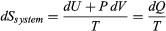for the working gas so that dSsystem + dSreservoir = 0. For any real process, less than the maximum work would be done (because of friction, for example), and so the actual amount of heat dQ? absorbed from the heat reservoir would be less than the maximum amount dQ. For example, the gas could be allowed to expand freely into a vacuum and do no work at all. Therefore, it can be stated that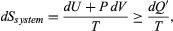with dQ? = dQ in the case of maximum work corresponding to a reversible process.

0 Dislike
Follow 2## Other Lessons for You

Kinematics Parameters
1.1 SOME DEFINITIONS (a) Distance: Distance between two points is the length of actual path travelled by the particle. It is a scalar quantity. Unit : m(metre) (b) Displacement: Displacement...PHYSICS
QUESTION Describe schematically the equipotential surfaces corresponding to (i) a constant electric field in the Z-direction, (ii) a field that uniformly increases in magnitude but remains in a constant...Anything could be understood, if learned in proper way, and by good coach.
Anything could be understood, if learned in proper way, and by good coach. Every body has there own way and pace of grasping things, so mentor/coach should be in sync (neither too fast nor too slow) with...### Looking for Class 12 Tuition ?

Learn from Best Tutors on UrbanPro.

Are you a Tutor or Training Institute?

Join UrbanPro Today to find students near you

X

### Looking for Class 12 Tuition Classes?

The best tutors for Class 12 Tuition Classes are on UrbanPro

• Select the best Tutor
• Book & Attend a Free Demo
• Pay and start Learning### Take Class 12 Tuition with the Best Tutors

The best Tutors for Class 12 Tuition Classes are on UrbanPro